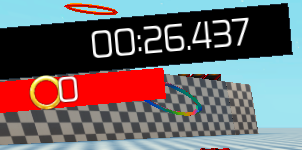# Ranking System with Stopwatch, need of insistence

Here’s the cheese. In this structure, when the player reaches the goal with the certain time, the player will be ranked with the what time tier that has been met.
… The stopwatch is being controlled through a GUI, and textbox. When scripting a ranking system, how can I get the calculations from the GUI?1 Like

Is there a string value inside the stopwatch? How does it work?

local player = game.Players.LocalPlayer
while game.Workspace:FindFirstChild(player.Name) == nil do
wait()
end

repeat wait(1) until game.Players.LocalPlayer.Character

local paused = false
local running = false
local begin = tick()
local pauseT = tick()
local current = 0
local timestart = false
local timer = script.Parent
local debounce = false

function display()
local minute = math.floor(current/60)
local minutes = (minute%60>=10 and “” or “0”)…tostring(minute%60)
local second = math.floor(current)
local seconds = (second%60>=10 and “” or “0”)…tostring(second%60)
local fraction = current%1
local milliseconds = ("%0.3f"):format(fraction):sub(3)
script.Parent.Time.Text = minutes…":"…seconds…"."…milliseconds
end

function startTimer(resetT)
running,paused = true,false
if (resetT) then current = 0 begin = tick() end
while (running) do
if (not paused) then
current = (tick()-begin)
display()
end
wait()
end
end

player.Character.Humanoid.Touched:connect(function(part)

``````if part.Name == "starterline" and not debounce then
timestart = true
debounce = true
if timestart == true then
local diff = (tick()-pauseT)
begin = (begin+diff)
startTimer(false)
wait(0.001)
debounce = false
end
end
``````

end)

Its a basic stopwatch script that’s been modify for a global trigger.